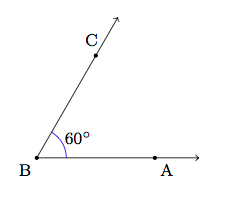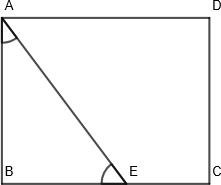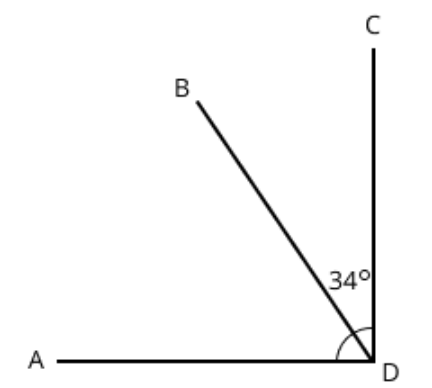# Shapes and Angles

## Objective

Solve problems involving the measure of two adjacent angles.

## Common Core Standards

### Core Standards

?

• 4.MD.C.7 — Recognize angle measure as additive. When an angle is decomposed into non-overlapping parts, the angle measure of the whole is the sum of the angle measures of the parts. Solve addition and subtraction problems to find unknown angles on a diagram in real world and mathematical problems, e.g., by using an equation with a symbol for the unknown angle measure.

?

• 1.OA.D.8

## Criteria for Success

?

1. Understand that angle measure is additive (i.e., when an angle is decomposed into non-overlapping parts, the angle measure of the whole is the sum of the angle measures of the parts).
2. Understand that adjacent angles are angles that share a vertex and a side.
3. Solve addition and subtraction problems to find unknown angles on a diagram in real-world and mathematical problems that involve two adjacent angles, e.g., by using an equation with a symbol for the unknown angle measure (MP.2, MP.4).
4. Write an equation to represent the unknown angle measure in a compound angle (MP.2).
5. Know the angle measure of common angles (a straight angle, a right angle) and use that knowledge to solve for missing angle lengths (MP.3).

## Tips for Teachers

?

### Lesson Materials

• Square-sized paper (2 per student)
• 180° Protractor (1 per student)

### Remote Learning Guidance

If you need to adapt or shorten this lesson for remote learning, we suggest prioritizing Anchor Task 2 (benefits from worked example). You might decide to facilitate this Anchor Task and Lesson 13's Anchor Task 2 before assigning Problem Sets from either lesson. Find more guidance on adapting our math curriculum for remote learning here.

#### Fishtank Plus

• Problem Set
• Student Handout Editor
• Vocabulary Package

?

### Problem 1

1. Take a square piece of paper and fold it along the diagonal. What is the measure of each of the angles created by the fold at each corner? What is the measure of the angle of the whole corner?
2. Take another square piece of paper and fold it so that there is a crease from one corner to another point on an opposite side that is not the diagonal corner.

#### References

EngageNY Mathematics Grade 4 Mathematics > Module 4 > Topic C > Lesson 10Concept Development Problem 1

Grade 4 Mathematics > Module 4 > Topic C > Lesson 10 of the New York State Common Core Mathematics Curriculum from EngageNY and Great Minds. © 2015 Great Minds. Licensed by EngageNY of the New York State Education Department under the CC BY-NC-SA 3.0 US license. Accessed Dec. 2, 2016, 5:15 p.m..

Modified by Fishtank Learning, Inc.

### Problem 2

1. Draw an angle that measures 60 degrees like the one shown here:1. Draw another angle that measures 25 degrees. It should have the same vertex and share side $\overrightarrow{BA}$.
2. How many angles are there in the figure you drew? What are their measures?
3. Make a copy of your 60 degree angle. Draw a different angle that measures 25 degrees and has the same vertex and also shares side $\overrightarrow{BA}$.
4. How many angles are there in the figure you drew? What are their measures?

#### References

Illustrative Mathematics Measuring Angles

Measuring Angles, accessed on March 7, 2018, 1:52 p.m., is licensed by Illustrative Mathematics under either the CC BY 4.0 or CC BY-NC-SA 4.0. For further information, contact Illustrative Mathematics.

### Problem 3

In the figure, $ABCD$ is a rectangle.1. $\angle EAB=37^\circ$ Find $\angle DAE$.
2. $\angle AEB=53^\circ$.  Find $\angle CEA$.

#### References

Illustrative Mathematics Finding an Unknown Angle

Finding an Unknown Angle, accessed on Feb. 20, 2019, 12:40 p.m., is licensed by Illustrative Mathematics under either the CC BY 4.0 or CC BY-NC-SA 4.0. For further information, contact Illustrative Mathematics.

Modified by Fishtank Learning, Inc.

## Problem Set & Homework

### Discussion of Problem Set

• For #1–4, why is it important to know that we are starting with a right angle or a straight angle?
• For #5, why is it important to know that ACDE is a rectangle?
• What was different about #6 compared to the other problems on the Problem Set?
• What new (or significant) math vocabulary did we use today to communicate precisely?

?

${\angle ADC}$ is a right angle.a.   Which of the following equations can be used to find the measure of $\angle ADB$?

1. a.   ${34+z=90}$

2. b.   ${z-34=90}$

3. c.   ${34\times z=90}$

4. d.   ${90\div34=z}$

b.   What is the measure, in degrees, of ${{\angle ADB}}$?

#### Mastery Response